Function Repository Resource:

# EinsteinSummation

Given tensors and their indices, sum over repeated indices

Contributed by: Carl Woll
 ResourceFunction["EinsteinSummation"][indices,tensors] sums over repeated indices of the tensors. ResourceFunction["EinsteinSummation"][indices→out,tensors] transposes the output if necessary so that the output tensor indices match out.

## Details and Options

The indices can be any expression.
ResourceFunction["EinsteinSummation"] accepts any type of tensor, either symbol or explicit, including any type of array.
On explicit rectangular arrays of scalars, ResourceFunction["EinsteinSummation"] will return a rectangular array. On symbolic tensors, ResourceFunction["EinsteinSummation"] will return a TensorContract object, possible wrapped in TensorTranspose.
If assumptions are given for symbolic tensors, then the specified indices must match the rank of the associated symbolic tensor.
Indices repeated more than twice are not supported.
Output indices can be specified, in which case the output tensor indices will be transposed to match the desired output.
If output indices are specified, they must match the tensor rank of the output.

## Examples

### Basic Examples (4)

Two explicit matrices:

 In:=Contract the second indices of the two matrices:

 In:=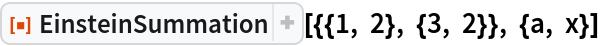Out=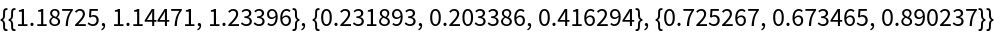Check:

 In:=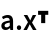Out=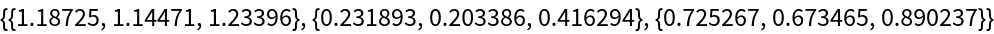Perform the contraction AikMjk for symbolic tensors A and M:

 In:=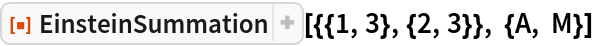Out=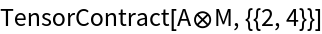### Scope (2)

Perform the contraction AikMjk and transpose the output:

 In:=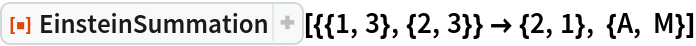Out=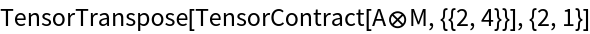The indices can be any expression:

 In:=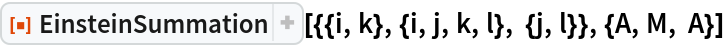Out=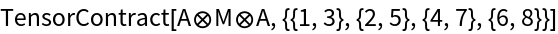### Possible Issues (2)

The number of index specifications must match the number of tensors:

 In:=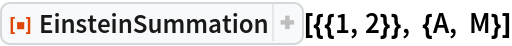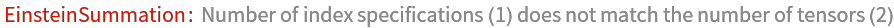Out=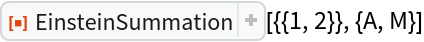If \$Assumptions is used to define symbolic tensors, the number of indices must match the tensor rank:

 In:=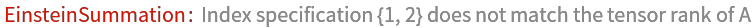Out=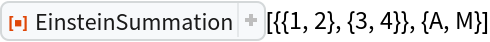Carl Woll

## Version History

• 1.0.0 – 16 October 2019